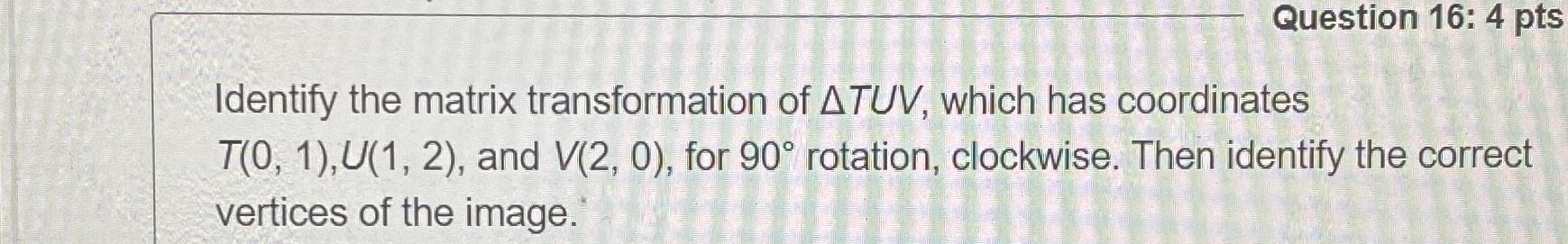### ¿Todavía tienes preguntas de matemáticas?

Pregunte a nuestros tutores expertos
Trigonometry
PreguntaIdentify the matrix transformation of $$\triangle T U V$$ , which has coordinates

$$T ( 0,1 ) , U ( 1,2 )$$ , and $$V ( 2,0 )$$ , for $$90 ^ { \circ }$$ rotation, clockwise. Then identify the correct vertices of the image.